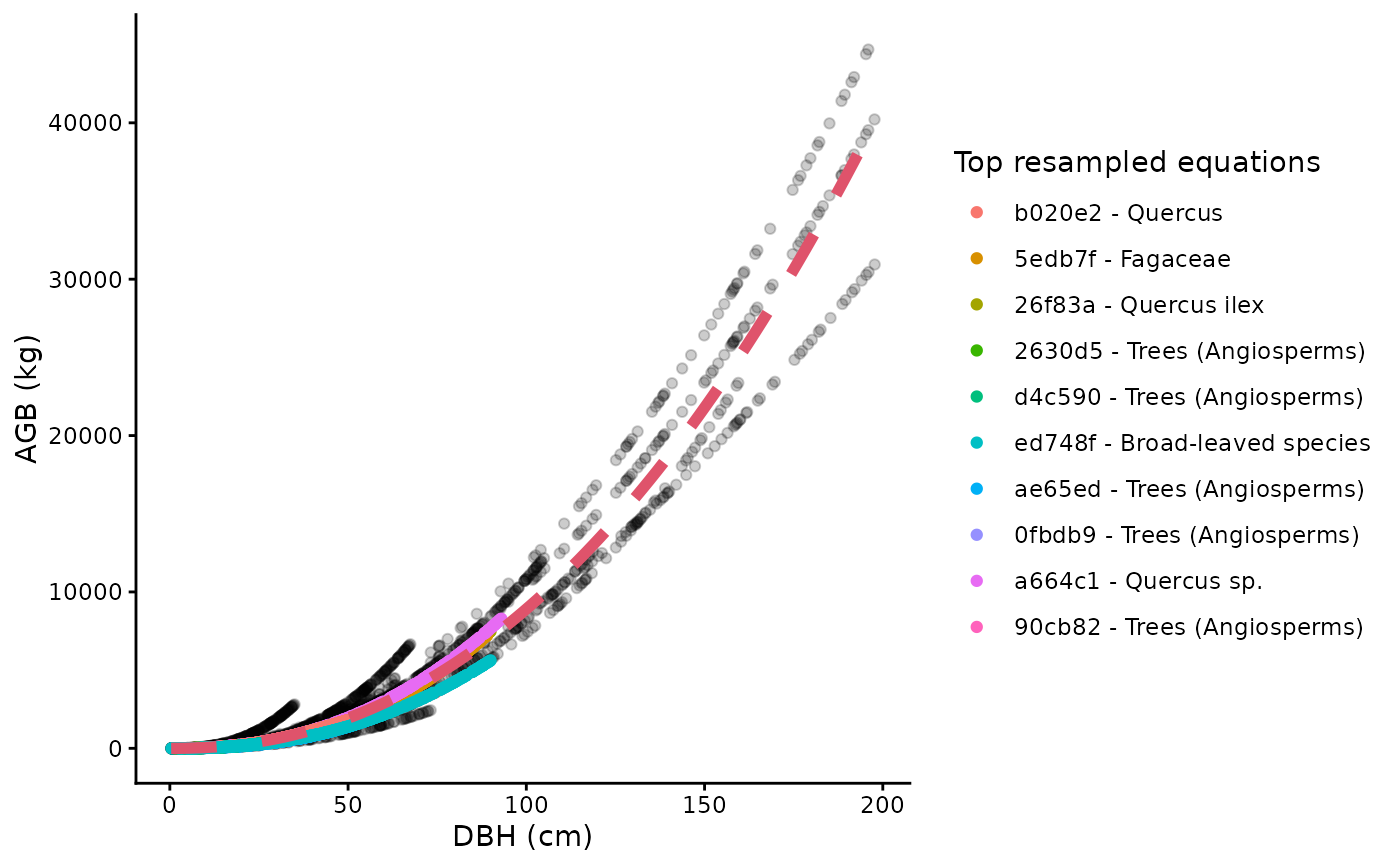This function illustrates the resampling of AGB values used in allodb. It creates objects of class "ggplot".

## Usage

``````illustrate_allodb(
genus,
coords,
species = NULL,
new_eqtable = NULL,
logxy = FALSE,
neq = 10,
eqinfo = "equation_taxa",
wna = 0.1,
w95 = 500,
nres = 10000
)``````

## Arguments

genus

A character value, containing the genus (e.g. "Quercus") of the tree.

coords

A numeric vector of length 2 with longitude and latitude.

species

A character value, containing the species (e.g. "rubra") of the tree. Default is `NULL`, when no species identification is available.

new_eqtable

Optional. An equation table created with the `new_equations()` function. Default is the base allodb equation table.

logxy

Logical: should values be plotted on a log scale? Default is `FALSE`.

neq

Number of top equations in the legend. Default is 10, meaning that the 10 equations with the highest weights are shown in the legend.

eqinfo

Which column(s) of the equation table should be used in the legend? Default is "equation_taxa".

wna

a numeric vector, this parameter is used in the `weight_allom()` function to determine the dbh-related and sample-size related weights attributed to equations without a specified dbh range or sample size, respectively. Default is 0.1.

w95

a numeric vector, this parameter is used in the `weight_allom()` function to determine the value at which the sample-size-related weight reaches 95% of its maximum value (max=1). Default is 500.

nres

number of resampled values. Default is "1e4".

## Value

An object of class "ggplot" showing all resampled dbh-agb values. The top equations used are shown in the legend. The red curve on the graph represents the final fitted equation.

`weight_allom()`, `new_equations()`.
``````illustrate_allodb(